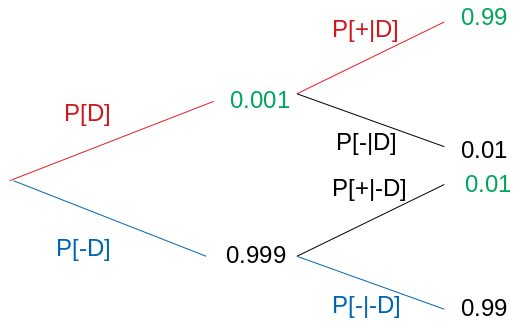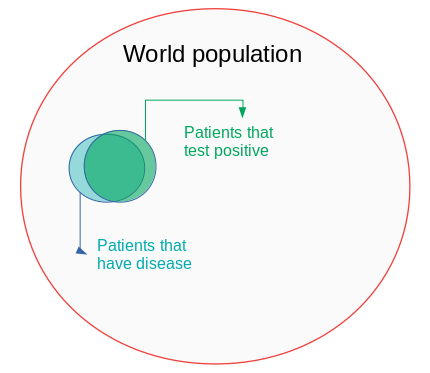# The Bayesian Thinking - I

Friday, June 8, 2018

A disease has affected 0.1% of the world’s population. The test for the disease correctly identifies 99% of people who have the disease and only incorrectly identifies 1% of people who don’t have the disease.
If you took the test and it showed a positive result, what is the probability that you have the disease?

It might seem that you have high chances of having the disease. But, it might not be so. Let’s see why. You can solve this problem using the Bayes theorem.

The Bayes theorem works on the concept of conditional probability i.e. the probability of occurrence of an event, given that some other event has already occurred.

The conditional probability of occurrence of event A, given B has already occurred is defined as:

$P[A \mid B] = \frac{P[A ∩ B]}{P[B]}$

The Bayes theorem is

$P[Cause \mid Evidence] = \frac{P[Evidence \mid Cause].P[Cause]}{P[Evidence]}$

The goal is to calculate the posterior conditional probability distribution of each of the possible unobserved causes given the observed evidence , i.e. P[Cause|Evidence].
However, in practice we are often able to obtain only the converse conditional probability distribution of observing evidence given the cause, P[Evidence|Cause].

Here, P[Cause], the probability of the cause, is often the most difficult ot find. It is known as prior probability. If our approach is that 0.1% of the population has the disease, and that’s of the general population. But the general population is not being tested. We assume, don’t we, that people are randomly tested whether they show symptoms or not, but in reality if you are tested then there’s an increased likelihood that you have it, so the 0.1% might not apply to patients actually being tested.

Coming back to our question, we want to find out P[D|+].
We’re given P[D] = 0.1%. We also know sensitivity of the test (True positive rate i.e. positive if disease) P[+|D] = 99%. The False positive rate (1 - specificity) is also known, P[+|-D] = 1%.Hence, using

$P[D \mid +] = \frac{P[+ \mid D].P[D]}{P[+]}$

we get, P[D|+] = 0.09 i.e. you have 9% chances of having the disease. It can be better understood with the help of the following venn diagram.## Bayesian statistical view of probability

The Bayesian view of probability is based on the degree of belief. In Bayesian statistics, probability is orderly opinion, and inference from data is nothing other than the revision of such opinion in the light of relevant new information.

In machine learning, the Bayes theorem is the idea behind a classification algorithm Naive Bayes, which is used in spam filters.

[Read the next part: The Bayesian Thinking - II]

References: